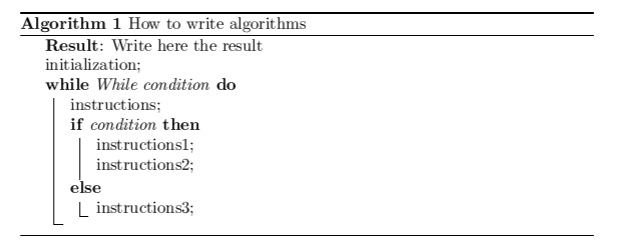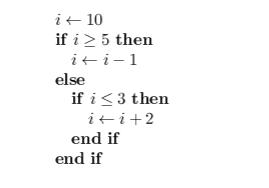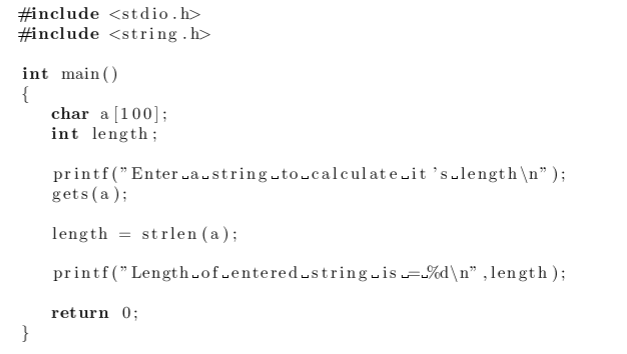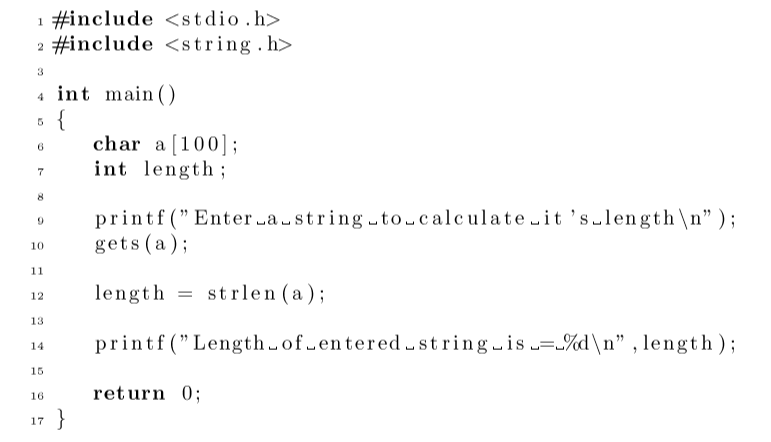Showing first {{hits.length}} results of {{hits_total}} for {{searchQueryText}}{{hits.length}} results for {{searchQueryText}}

Ingen Søge Resultater

To create algorithms in Latex you can use algorithm2e, algorithmic or Listings environment. Contents 1 Algorithm2e package 2 Algorithmic package 3 Listings package 3.1 Languages supported Algorithm2e package \usepackage[options ]{algorithm2e} Example \usepackage[ruled,vlined]{algorithm2e} ... \begin{algorithm}[H] \SetAlgoLined \KwResult{Write here the result } initialization\; \While{While condition}{ instructions\; \eIf{condition}{ instructions1\; instructions2\; }{ instructions3\; } } \caption{How to write algorithms} \end{algorithm}Algorithmic package \usepackage{algorithmic} Example \begin{algorithmic} \STATE $i\gets 10$ \IF {$i\geq 5$} \STATE $i\gets i-1$ \ELSE \IF {$i\leq 3$} \STATE $i\gets i+2$ \ENDIF \ENDIF \end{algorithmic}Listings package \usepackage{listings} After \begin{document} put \lstset{language=Pascal} to set your language; or you can specify the language while including the file with the following command: \lstinputlisting[language=C]{source.c} Languages supported ABAP IDL Plasm ACSL inform POV Ada Java Prolog Algol JVMIS Promela Ant ksh Python Assembler Lisp R Awk Logo Reduce bash make Rexx Basic Mathematica1 RSL C Matlab Ruby C++ Mercury S Caml MetaPost SAS Clean Miranda Scilab Cobol Mizar sh Comal ML SHELXL csh Modula-2 Simula Delphi MuPAD SQL Eiffel NASTRAN tcl Elan Oberon-2 TeX erlang OCL VBScript Euphoria Octave Verilog Fortran Oz VHDL GCL Pascal VRML Gnuplot Perl XML Haskell PHP XSLT HTML PL/I Example : \lstinputlisting[language=c]{c.c}Line numbers are available for all displayed listings, e.g. tiny numbers on the left, each second line, with 5pt distance to the listing: \lstset{numbers=left, numberstyle=\tiny, stepnumber=1, numbersep=5pt}